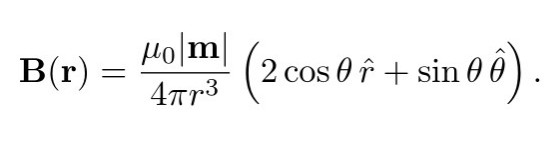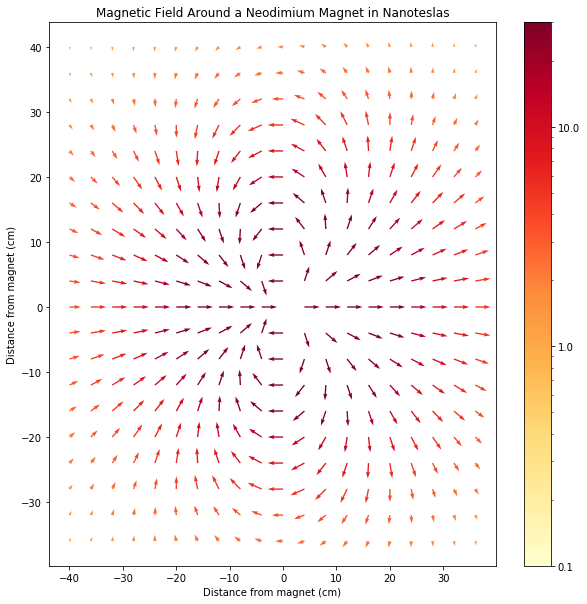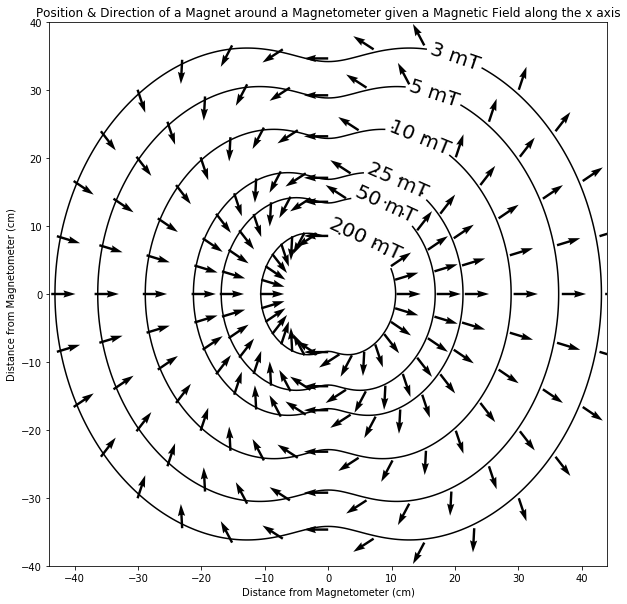Modeling a Magnetic Field

Modeling the Magnetic Field around a Magnet

This week, I modeled a magnetic field around a magnet. The strength and direction of a magnetic field can be modeled by the following polar equation where θ is the angle from the direction of the magnetic north pole, r is the distance from the magnet, and |m| is the strength of the rare earth magnet.Since the magnetic field is only dependent on r and,it can be modeled in two dimensions. This would mean that the graph could be rotated around the x axis, and the magnetic field would not change. Below is a model of the magnet in the x, y plane, where the magnetic North pole is pointed in the positive x direction. The arrows indicate the direction of the magnetic field at a certain distance from the magnet, and the strength of the magnetic field (in nanoteslas) is indicated by the color, with purple being a field strength of 30 nanoteslas and over.As we can see, the magnetic field close to the magnet is strong, but quickly decreases. This is because the strength of the magnetic field has a cubic relationship with respect to the distance from the magnet.

Modeling the Position of a Magnet around a Magnetometer

Next, I created a model of where the magnet could be located relative to a reading of the magnetometer. In the following chart, the lines show all possible locations of a magnet if a magnetometer reads a magnetic field of a certain strength that points in the positive x direction. The arrows show the direction which the magnet’s north pole needs to be facing, if it was at a specific position on the line.On the shoulder sensor, the magnetometer will not read the magnetic field pointing in the positive x direction, however knowing that a magnetic field points in a specific direction, we can orientate the chart so that the magnetic field points in the x direction. Similar to the magnetic field, this chart can be rotated around the x axis for a 3d model since the angle between the magnetometer and the distance between the magnet and the magnetometer would not change if the magnet was rotated around the x axis.

1.Michael Parker says: